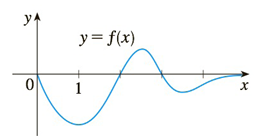Chapter 3.9, Problem 47E

Chapter
Section
Textbook Problem

# The graph of a function is shown in the figure. Make a rough sketch of an antiderivative F, given that F ( 0 ) = 1 .To determine

To make:

A rough sketch of an antiderivative F(x).

Explanation

Explanations:

1) Concept:

The function is increasing if f'x>0 and decreasing if f'x<0.

2) Given:

F(0)=1

3) Calculation:

Since F(0)=1, (0, 1) is a point of F(x). Start from the point (0,1).

fx is negative when x0,2. Therefore, the F(x) is decreasing from (0, 1).

Notice f0=f2=0. So, F has horizontal tangent when x=0 & x=2.

For 2<x<3, f(x) is positive and increasing

### Still sussing out bartleby?

Check out a sample textbook solution.

See a sample solution

#### The Solution to Your Study Problems

Bartleby provides explanations to thousands of textbook problems written by our experts, many with advanced degrees!

Get Started

#### Simplify: 7296

Elementary Technical Mathematics

#### True or False: is a rational function.

Study Guide for Stewart's Single Variable Calculus: Early Transcendentals, 8th

#### What is the goal of a single-case experimental research design?

Research Methods for the Behavioral Sciences (MindTap Course List)